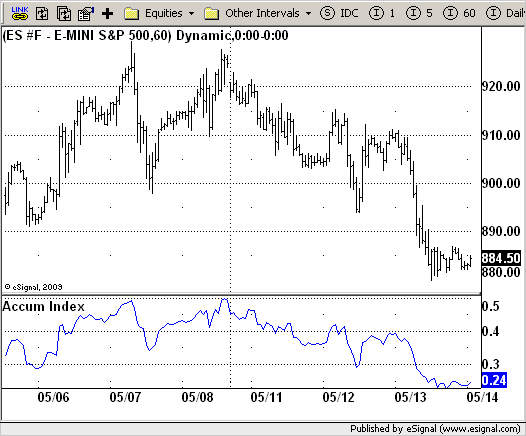# Accumulation Swing Index (ASI)

ICE Data Services -

 AccumSwingIndex.efs EFSLibrary - Discussion Board

File Name: AccumSwingIndex.efs

Description:
Accumulation Swing Index (ASI)

Formula Parameters:
Daily Limit : 10000

Notes:
The Accumulation Swing Index is a cumulative total of the Swing Index.
The Accumulation Swing Index was developed by Welles Wilder.
The SwingIndex function was developed to help cut through the maze of
Open, High, Low and Close prices to indicate the real strength and direction
of the market. The Swing Index function looks at the Open, High, Low and
Close values for a two-bar period. The theory is that there are four cross-bar
and one intra-bar comparisons that are strong indicators of an up or down day.
The Swing Index returns a number between -100 and 100. If the factors point toward
an up day, then the function value will be positive and vice versa. In this way,
the Swing Index gives us definite short-term swing points, and it can be used to
supplement other methods as a breakout indicator. A breakout is indicated when the
value of the Accumulation Swing Index (ASI) exceeds the ASI value on the day when a
previous significant High Swing Point was made. A downside breakout is indicated when
the value of the ASI drops below the ASI value on a day when a previous significant
low swing point was made.
Since only futures have a relative daily limit value, this function only makes sense
when applied to a futures contract. If you use this function and it only plots a zero
flat line, check the Daily Limit value.`/*********************************Provided By: eSignal (Copyright c eSignal), a division of Interactive Data Corporation. 2009. All rights reserved. This sample eSignal Formula Script (EFS) is for educational purposes only and may be modified and saved under a new file name. eSignal is not responsible for the functionality once modified. eSignal reserves the right to modify and overwrite this EFS file with each new release.Description: Accumulation Swing Index (ASI) Version: 1.0 05/13/2009 Formula Parameters: Default: Daily Limit 10000 Notes: The Accumulation Swing Index is a cumulative total of the Swing Index. The Accumulation Swing Index was developed by Welles Wilder. The SwingIndex function was developed to help cut through the maze of Open, High, Low and Close prices to indicate the real strength and direction of the market. The Swing Index function looks at the Open, High, Low and Close values for a two-bar period. The theory is that there are four cross-bar and one intra-bar comparisons that are strong indicators of an up or down day. The Swing Index returns a number between -100 and 100. If the factors point toward an up day, then the function value will be positive and vice versa. In this way, the Swing Index gives us definite short-term swing points, and it can be used to supplement other methods as a breakout indicator. A breakout is indicated when the value of the Accumulation Swing Index (ASI) exceeds the ASI value on the day when a previous significant High Swing Point was made. A downside breakout is indicated when the value of the ASI drops below the ASI value on a day when a previous significant low swing point was made. Since only futures have a relative daily limit value, this function only makes sense when applied to a futures contract. If you use this function and it only plots a zero flat line, check the Daily Limit value. **********************************/var fpArray = new Array();var bInit = false;function preMain() { setPriceStudy(false); setShowCursorLabel(true); setShowTitleParameters( false ); setStudyTitle("Accum Index"); setCursorLabelName("Accum Index"); var x = 0; fpArray[x] = new FunctionParameter("DailyLimit", FunctionParameter.NUMBER); with(fpArray[x++]) { setName("Daily Limit"); setLowerLimit(1); setDefault(10000); } }var xAccumIndex = null;function main(DailyLimit) {var nAccumIndex = 0; if (bInit == false) { xAccumIndex = efsInternal("Calc_ASI", DailyLimit); bInit = false; } nAccumIndex = xAccumIndex.getValue(0); if (nAccumIndex == null) return; return nAccumIndex;}var xOpen = null;var xClose = null;function Calc_ASI(nDailyLimit) {var K = 0;var R = 0;var AbsHighClose = 0;var AbsLowClose = 0;var AbsCloseOpen = 0;var SwingIndex = 0;var ASI = ref(-1); if (xOpen == null) xOpen = open(); if (xClose == null) xClose = close(); var h0 = high(0); var l0 = low(0); var o0 = xOpen.getValue(0); var o1 = xOpen.getValue(-1); var c0 = xClose.getValue(0); var c1 = xClose.getValue(-1); if (c1 == null) return; AbsHighClose = Math.abs(h0 - c1); AbsLowClose = Math.abs(l0 - c1); AbsCloseOpen = Math.abs(c1 - o1); if (AbsHighClose >= AbsLowClose){ K = AbsHighClose; if (AbsHighClose >= (h0 - l0)) R = AbsHighClose - 0.5 * AbsLowClose + 0.25 * AbsCloseOpen; else R = (h0 - l0) + 0.25 * AbsCloseOpen; } else { K = AbsLowClose; if (AbsLowClose >= (h0 - l0)) R = AbsLowClose - 0.5 * AbsHighClose + 0.25 * AbsCloseOpen; else R = (h0 - l0) + 0.25 * AbsCloseOpen; } if (R != 0) SwingIndex = 50 * (((c0 - c1) + 0.50 * (c0 - o0) + 0.25 * (c1 - o1)) / R ) * K / nDailyLimit; else SwingIndex = 0; ASI += SwingIndex; return ASI;}`